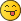Dat file line type 1 explanation - Printable Version +- LDraw.org Discussion Forums (https://forums.ldraw.org) +-- Forum: LDraw Programs (https://forums.ldraw.org/forum-7.html) +--- Forum: LDraw Editors and Viewers (https://forums.ldraw.org/forum-11.html) +--- Thread: Dat file line type 1 explanation (/thread-24178.html) Dat file line type 1 explanation - Cam's Bricks - 2020-08-25 Hello, I am trying to write some lines to scale a part in the PLI in Lpub3D. If I have it correct, I need to use the scaling functions in the line for this part. I read the article here https://www.ldraw.org/article/218.html but the only given parts are the x,y,z coordinates as the first 3 characters.  Can someone explain what the rest of the numbers do? I know some are a scale, some are rotation, and I *think* that some are a repeat but I have no idea.  1 x y z a b c d e f g h i I am looking at "a b c d e f g h i" in case my post makes no sense.  Thanks, Cam PS if I am barking up the wrong tree, please help me understand. I have a custom part that renders MUCH to large in the PLI in Lpub3D and I would like it to show at a smaller size. RE: Dat file line type 1 explanation - Orion Pobursky - 2020-08-25 It's a 3D transformation matrix. There's a linked tutorial in the last paragraph of the Type 1 line section. RE: Dat file line type 1 explanation - N. W. Perry - 2020-08-25 (2020-08-25, 5:06)Cam\s Bricks Wrote: Hello, I am trying to write some lines to scale a part in the PLI in Lpub3D. If I have it correct, I need to use the scaling functions in the line for this part. I read the article here https://www.ldraw.org/article/218.html but the only given parts are the x,y,z coordinates as the first 3 characters.  Can someone explain what the rest of the numbers do? I know some are a scale, some are rotation, and I *think* that some are a repeat but I have no idea.  1 x y z a b c d e f g h i I am looking at "a b c d e f g h i" in case my post makes no sense.  Thanks, Cam PS if I am barking up the wrong tree, please help me understand. I have a custom part that renders MUCH to large in the PLI in Lpub3D and I would like it to show at a smaller size. Short answer: To simply scale a non-rotated part, you're just concerned with a, e and i. For an unscaled, non-rotated part, these are all 1 (and everything else is 0). To make the part smaller, change these three values equally to something less than 1 (e.g. 0.5 for 50% scaling). Longer answer: All of those values are actually both rotation and scaling. They mathematically describe the orientation and length of the part's X, Y and Z vectors with respect to the global X, Y and Z vectors. The explanation that most helped me understand was this one from Roland: Quote:The columns in the LDCad dialog indicate the direction of the part's local xyz axis' as seen from absolute space. First column indicates the vector of the X-ax's (1,0,0 as it's non rotated). 2nd the Y-axis and 3rd the z-axis. Normally all these vectors are normalized (length == 1.0), with any other length scaling will be applied. You could also read it from a row's perspective to go the other way (direction of world axis' as seen from local part space) When you read a type 1 line, you're looking at it the second way—from the part's perspective. So the a, b, c values are looking along the part's X axis; d, e, f are looking along its Y axis, and g, h, i are looking along its Z axis. You could mentally read it like this: value a equals "the X of X", b equals "the Y of X", d equals "the X of Y", h equals "the Y of Z", and so on. So let's say you have a matrix like this:  Code:`0 0 1 0 1 0 -1 0 0` The first three values, 0 0 1, tell you what you see if you look down the part's X axis. The "X of X" is 0, the "Y of X" is 0, and the "Z of X" is 1, meaning that when you look down the part's X (positive) axis, you see the global Z axis (also positive). For the second triplet, you see that the Y axis is unchanged—the "Y of Y" is 1. For the last three values, you look down the part's Z axis and see the global X axis, this time in the negative direction. A good way to visualize this in LDCad is to select a rotated part and choose relative orientation. Look at the editing compass (be sure the preference is set to also display global axes) and compare the direction of the X, Y and Z arrows. If everything's at simple right angles, you can see how the matrix values relate to the orientation pretty easily. Now, when you rotate by non-right angles, or combine rotation and scaling, that's when things get hairy.RE: Dat file line type 1 explanation - Cam's Bricks - 2020-08-25 Thank you so much!! That really helps!